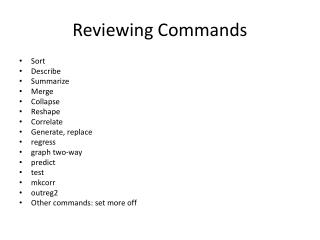Download PresentationReviewing Commands

# Reviewing Commands - PowerPoint PPT PresentationDownload Presentation## Reviewing Commands

- - - - - - - - - - - - - - - - - - - - - - - - - - - E N D - - - - - - - - - - - - - - - - - - - - - - - - - - -
##### Presentation Transcript

1. Reviewing Commands • Sort • Describe • Summarize • Merge • Collapse • Reshape • Correlate • Generate, replace • regress • graph two-way • predict • test • mkcorr • outreg2 • Other commands: set more off

2. Debriefing the database: Mobile

3. Debriefing the Database • What went wrong along the way? • Code mismatch on polcon • Do file won’t run • Creating operator count variable • Missing data • Source: WDI, polcon, operator db • Other?

4. Linear Regression Y = Xβ + ε βOLS = (X’X)-1X’Y • X’Y = X’Xβ + X’ε • X’ε = 0 by assumption  β = (X’X)-1X’Y

5. Why linear regression? • Good foundation for thinking about all analysis. • criteria for estimators • unbiased: E(β*) = β • efficient: σ2(β*) < σ2(β) • asymptotic properties: plim β* • montecarlo studies for small sample properties • maximum likelihood estimation • given a population distribution, which parameters of the distribution best match the observed data? • For normal error term, βMLE = βOLS • R2 • error term • Many of the problems we discuss in regression are found in the assumptions concerning the error term: probability distribution, variance, correlation ^

6. Assumptions of the Classic Linear Model

7. Linear Regression & Causality • Define endogeneity: “When there is correlation between a regressor and the error term, that regressor is said to be endogenous” • Measurement error in explanatory variables • Autoregression (lagged variable as predictor) • Simultaneity/Reverse causality • Omitted variable • Sample Selection & unobserved heterogeneity • Missing data • Groups

8. More general frameworks build from the linear model • (feasible) Generalized Least Squares: GLS or fGLS • Weighted least squares with sample variance/covariance as the weighting matrix • reg3 or xtgls • Generalized Linear Model: GLM • g{E(y)} = xβ, y ~ F • g{} is the link function • F is the distribution family • Classical model with normal errors: • g{} is identity & y ~ Normal • Alternatives: • g{}: logarithmic, logit, probit, complementary log-log, negative binomial • F: normal, binomial, poisson, negative binomial, gamma • glm or xtgee

10. Missing Data • Summarize • Compare: pick most incomplete variable • Take a relatively complete descriptive variable, such as pop or GDP • Test if mean is different for observations where the incomplete variable is defined and missing • Sort & browse • Examine observations for differences where the variable is missing

11. Categorical Variable • Where is the median stored? • Summarize polcon • r(p50) gives the median [r(N), r(mean), r(max), r(Var)] • gen polcon_hi = 0 • replace polcon_hi = 1 if polcon>r(p50) • Scatter mobile_subspolcon_hi • Why doesn’t this look great? • jitter • Add two lines: • Scatter mobile_subspolcon_hi || lfitmobile_subspolcon_hi • Scatter mobile_subspolcon_hi || lfitcimobile_subspolcon_hi

12. Graph quadratic fit & confidence intervals • Scatter mobile_subsgnipercap • Add a quadratic line • || qfitmobile_subsgnipercap • || qfitcimobile_subsgnipercap

13. Lagged variable • Start with wdi_mobile • Easy lag: redefine Y2001 as mobile_lag • Reshape long • Hard lag: often necessary • Sort id year • gen mobilesubs_lag = mobilesubs[_n-1] • keep if year==2002 • keep id mobilesubs_lag • merge into database

14. Regression • regress mobile_subsgdp pop gnipercaptelpolcon ops • graph residuals • rvfplot (vs. fitted), rvpplot (vs. predictor) • test for equal variance • estathettest • test for omitted variable • estatovtest • robust estimation: • “White-Huber heteroskedasticity-consistent estimator”, “sandwhich estimator” “White-washing the data” • regress <outcome variable> <explanatory variables>, vce(robust) • graph added effect of each variable • avplots

15. Post-estimation • Predict • Predict yhat • Estimates • store output for analysis, eg for hausman test • Test • simple and composite Wald tests • lrtest

16. Making tables • Correlation table • mkcorr • Regression table • outreg2